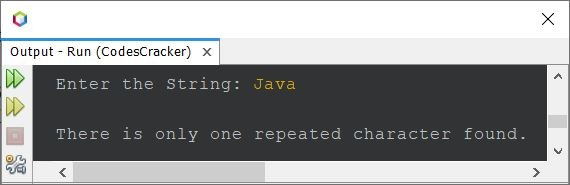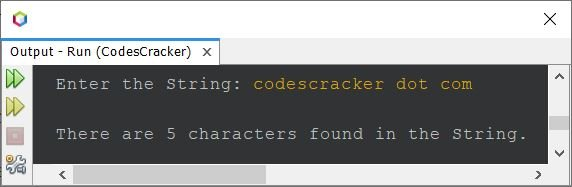# Java Program to Count the Number of Repeated Characters in a String

This article is created to cover a program in Java that counts and prints the number of repeated or duplicate characters available in a given string. For example, if the given string is "Java Programming", then the output will be 4. Because the characters a, r, g, and m are available more than once.

## Count the Number of Repeated Characters in a String: Basic Version

The question is: write a Java program to count the number of repeated characters in a string. The string must be received by the user at the runtime of the program. Here is its sample run:

```import java.util.Scanner;

public class CodesCracker
{
public static void main(String[] args)
{
String str;
char ch;
int strLen, i, count, j, k, repChars=0;
Scanner s = new Scanner(System.in);

System.out.print("Enter the String: ");
str = s.nextLine();

strLen = str.length();
char[] arr = new char[strLen];

for(i=0; i<strLen; i++)
arr[i] = str.charAt(i);

for(i=0; i<strLen; i++)
{
ch = arr[i];
count = 0;
for(j=(i+1); j<strLen; j++)
{
if(ch==arr[j])
{
count++;
for(k=j; k<(strLen-1); k++)
arr[k] = arr[k+1];
strLen--;
j--;
}
}
if(count>0)
repChars++;
}

System.out.println("\nTotal Number of Repeated Characters = " +repChars);
}
}```

The snapshot given below shows the sample run of the above program with user input codescracker as a string to count and print the total number of duplicate characters available in it:That is, the characters c, e, and r are the three characters available a repeated number of times. Therefore, the output was 3.

## Count the Number of Repeated Characters in a String: Complete Version

The problem with the above program is that if the user enters a string containing white spaces, then white space duplication also gets counted. Therefore, let's modify the above program. Some other modifications are also done in the program given below:

```import java.util.Scanner;

public class CodesCracker
{
public static void main(String[] args)
{
int i, count, j, k, repChars=0;
Scanner s = new Scanner(System.in);

System.out.print("Enter the String: ");
String str = s.nextLine();

str = str.replaceAll("\\s+", "");
str = str.toLowerCase();
int strLen = str.length();
char[] arr = new char[strLen];

for(i=0; i<strLen; i++)
arr[i] = str.charAt(i);

for(i=0; i<strLen; i++)
{
count = 0;
for(j=(i+1); j<strLen; j++)
{
if(arr[i]==arr[j])
{
count++;
for(k=j; k<(strLen-1); k++)
arr[k] = arr[k+1];
strLen--;
j--;
}
}
if(count>0)
repChars++;
}

if(repChars==0)
System.out.println("\nNo repeated character found.");
else if(repChars==1)
System.out.println("\nThere is only one repeated character found.");
else
System.out.println("\nThere are " +repChars+ " characters found in the String.");
}
}```

Here is its sample run with user input "Java" as a string:Here is another sample run with user input, "codescracker dot com"In the above program, the statement:

`str = str.replaceAll("\\s+", "");`

is used to remove all white spaces (single space, multiple space (tabs)). And the following statement:

`str = str.toLowerCase();`

is used to convert the whole string to lowercase. Because the same character in uppercase and lowercase gets treated as a different character.

Java Online Test

« Previous Program Next Program »